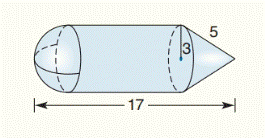Chapter 9.CR, Problem 19CR### Elementary Geometry for College St...

6th Edition
Daniel C. Alexander + 1 other
ISBN: 9781285195698

#### Solutions

Chapter
Section### Elementary Geometry for College St...

6th Edition
Daniel C. Alexander + 1 other
ISBN: 9781285195698
Textbook Problem
1 views

# The solid shown consists of a hemisphere (half of a sphere), a cylinder, and a cone. Find the exact volume of the solid.To determine

To find:

The exact volume of the solid.

Explanation

Formula used:

Volume of half sphere:

V=23πr3

Volume of cylinder:

V=πr2h

Volume of cone:

V=13πr2h

Given:

The solid shown consists of a hemisphere (half of a sphere) a cylinder and a cone.

Calculation:

The volume of given solid is,

V=volume of half sphere+ volume of cylinder+volume of coneV=23πr3+πr2h1+13πr2h2

First we find the height of the cone,

Right circular cone as shown in the above figure.

Also, the slant height is the hypotenuse of a right circular cone with legs equal to the lengths of the altitude of the cone and the radius of the base.

Applying the Pythagorean Theorem,

l2=r2+h2252=32+h2225=9+h22Interchanging the values and subtracting 9 on both sides,h22+99=259h22=16h2=16h2=4

The height of the cone is h2=4.

Next we find the height of the cylinder,

Already we know that, in the given solid the radius of cone is same as to the radius of half sphere.

Also, the radius of half sphere is same as to the height of the half sphere.

So, the height of the half sphere is h3=3

### Still sussing out bartleby?

Check out a sample textbook solution.

See a sample solution

#### The Solution to Your Study Problems

Bartleby provides explanations to thousands of textbook problems written by our experts, many with advanced degrees!

Get Started

#### In Problems 19-44, factor completely. 29.

Mathematical Applications for the Management, Life, and Social Sciences

#### In Exercises 7-28, perform the indicated operations and simplify each expression. 17. 1+1x11x

Applied Calculus for the Managerial, Life, and Social Sciences: A Brief Approach

#### Add and reduce to lowest terms.

Contemporary Mathematics for Business & Consumers

#### True or False: is monotonic.

Study Guide for Stewart's Multivariable Calculus, 8th